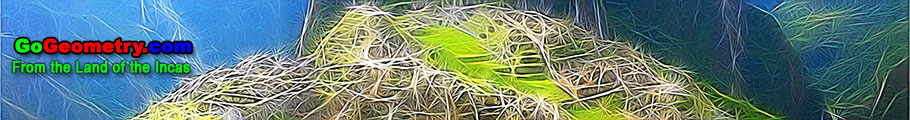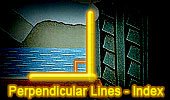Perpendicular Lines, Theorems and Problems - Table of Content  (18)Proposed Problem 204. Right Triangle, Incircle, Excircles, Inradius, Exradii, Perpendicular lines. Proposed Problem 203. Right Triangle, Excircles, Exradii, Hypotenuse, Perpendicular lines. Proposed Problem 202. Right Triangle, Incicrle, Excircles relatives to catheti, Points of Tangency, Exradius, Semiperimeter, Perpendicular lines. Proposed Problem 201. Right Triangle, Excircles, Points of Tangency, Exradius, Semiperimeter, Perpendicular lines. Proposed Problem 200. Right Triangle, Incircle, Excircles, Points of Tangency, Inradius, Perpendicular lines. Archimedes Arbelos and Square 2. Dynamic Geometry Software. Step-by-Step construction, Manipulation, and animation, Perpendicular lines. Archimedes Arbelos and Square 1. Dynamic Geometry Software. Step-by-Step construction, Manipulation, and animation, Perpendicular lines. Proposed Problem 179. Rhombus, Square, Diagonal, Angle, Perpendicular lines, Parallel lines. Proposed Problem 137. Orthic Triangle, Altitudes, Perpendicular, Incircle, Collinear Points, Parallelogram, Perpendicular lines. Proposed Problem 136. Orthic Triangle, Altitudes, Perpendicular lines, Concyclic Points. Proposed Problem 135. Orthic Triangle, Altitudes, Perpendicular, Perpendicular lines. Bottema's Theorem: Triangle and Squares with Interactive Geometry Software Step-by-Step construction, Manipulation, and animation. Perpendicular lines. Go to Page: Previous | 1 | 2 | 3 | 4 | 5 | 6 | 7 | 8 | 9 | 10 | 11 | 12 | 13 | 14 | 15 | 16 | 17 | 18 | 19 | 20 | Next